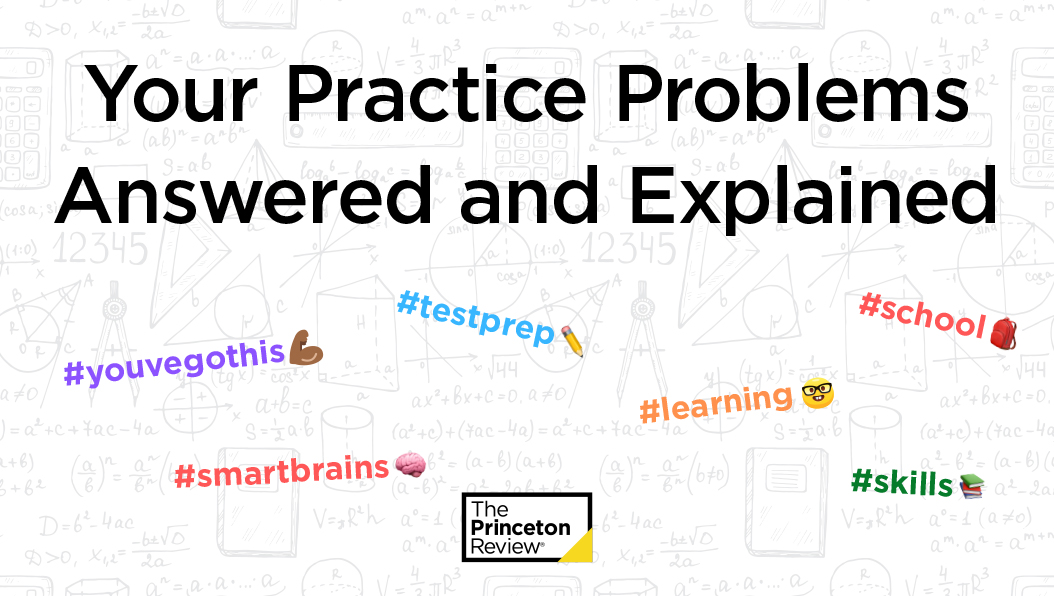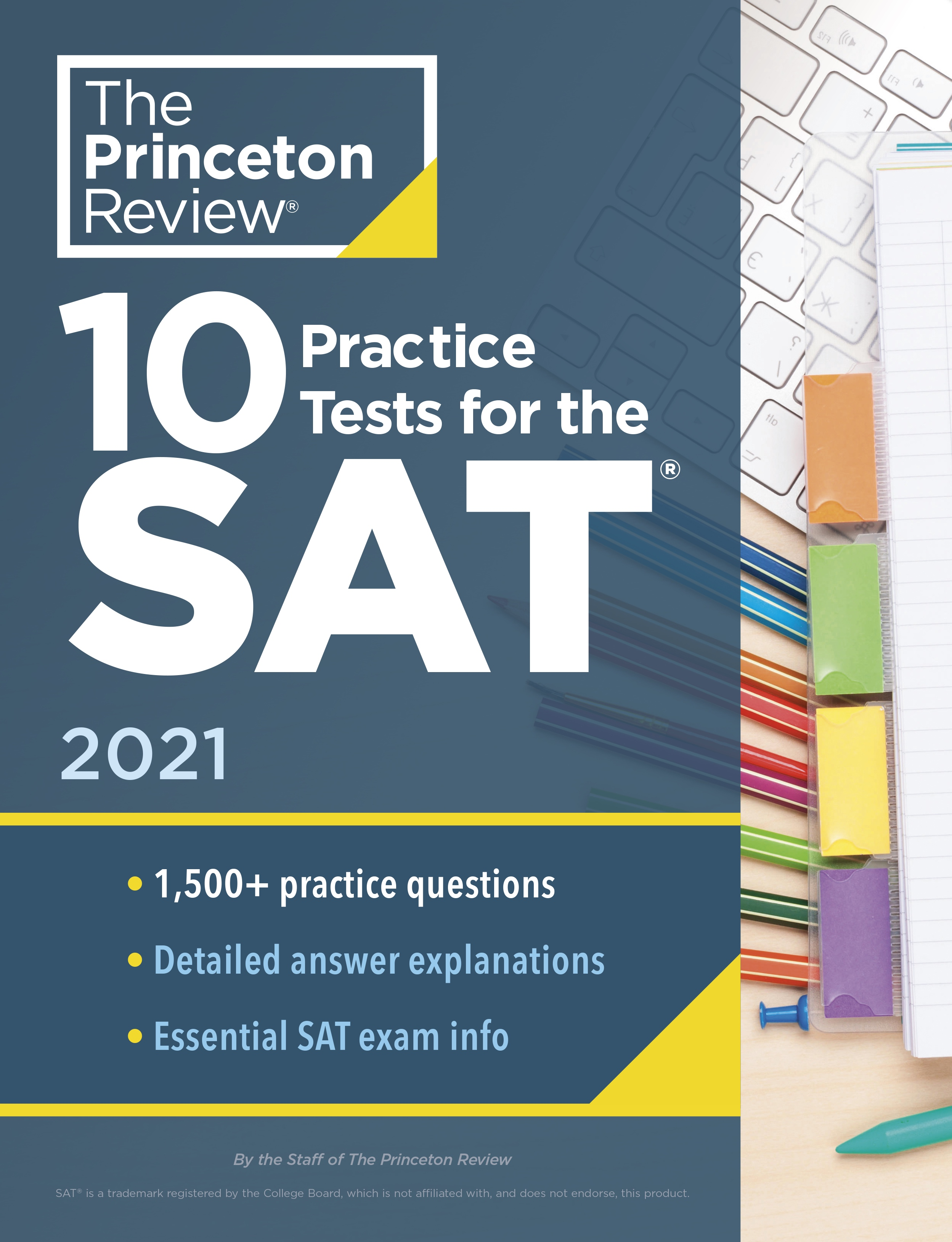We publish practice problems on our social media pages. Here's where you can find the correct answers—and explanations.

## 10/2/20

The question asks for a pair of equations to represent the situation. Translate each statement, piece by piece. The first part tells us that “the product of x and y is 76.” Since product means multiplication, the first equation must be xy = 76, so you can eliminate (C). The second part says that “x is twice the square of y,” which translates to x = 2y2, so eliminate (B) and (D), and (A) is the only choice left. Notice that only the y needs to be squared, which is why (B) is wrong. The second equation for (B) would be written as “the square of twice y,” which is not what the problem states. The correct answer is (A).

## 9/28/20

The question asks for the least possible value of 4r + 3. Notice that this question is asking for an expression instead of a variable, so manipulate the inequality so that you get 4r + 3 in the inequality. Treat each side of the inequality separately to avoid confusion. Starting with the –6 < –4r + 10 part, multiply both sides of the inequality by –1, remembering to switch the sign, to get 6 > 4r – 10. Add 13 to each side to get 19 > 4r + 3. Then solve the right side of the inequality. Again, multiply both sides of the inequality by –1, switching the sign to get 4r – 10 ≥ –2. Now add 13 to each side of the equation: 4r + 3 ≥ 11. Finally, combine the equations to get the range for 4r + 3. The least possible value of the expression is 11. If you see the answer before the last step above, you don’t need to combine the equations. The correct answer is (D).

## ***

### Practice makes perfect! Strengthen your skills with 10 Practice Tests for the SAT, available here and wherever books are sold.## 9/25/20

The question asks for the value of a in an equation with fractions. The lowest number that both 8 and 10 are factors of is 40. Convert the fractions to a denominator of 40: 5/40 + 4/40 = 9/40. There is no factor that 9 and 40 have in common, so the fraction cannot be reduced. The number in place of a in a/b is 9. Be careful not to choose (D), which contains the value of b. The correct answer is (B).

## 9/21/20

The question asks for the value of x in an equation with exponents. Negative exponents mean to take the reciprocal and apply the positive exponent. So 9–2= (1/9)2 = 1/81. Now find what power of 1/3 equals 1/81. Because 34 = 81, (1/3)4  = 1/81 , and x must be 4. The correct answer is (C).

## 9/18/20

The question asks for the value of an expression for a certain value of the variable. Plug in the number given for a in the expression to find the value: –2 + (–2)2 – (–2)3 + (–2)4 – (–2)5. Remember PEMDAS, the order of operations: The first thing to do here is deal with the Exponents, then we can take care of the Addition and Subtraction: –2 + 4 – (–8) + 16 – (–32), which simplifies to –2 + 4 + 8 + 16 + 32 = 58. The correct answer is (D).

## 9/14/20

The question asks for the value of n. First, simplify the equation 4x • n2 = 4+ 1 • n to 4x • n = 4+ 1, and then try an easy number for x. If x = 2, then 42 • n = 42 + 1. Since 16n = 43, then 16n = 64 and n = 4. The correct answer is (B).

## 9/11/20

The question asks for the value of t. To figure out how much you need to add to 2.74 to get to 4.22, take 4.22 – 2.74 on your calculator. The difference between the two numbers is 1.48. This increase reflects the same number, t, added to each of the four numbers on the list. Divide 1.48 by 4 to find that t = 0.37. The correct answer is (D).

## 9/7/20

The question asks for the value of 24/x . First, solve for x. Divide both sides of the equation by 3, and you get x = 4. Then divide 24 by 4, which gives you 6. The correct answer is (D).

## 9/4/20

The zero of is the value of the variable, in this case x, when the equation is set to 0. This is also called the root or solution of an equation. Set the equation to 0 to get 0 = 2x2 – dx – 6. Plug 6 in for to get 0 = 2(62) – d(6) – 6. Simplify the equation to get 0 = 72 – 6– 6, or 0 = 66 – 6d. Solve for to get –66 = –6d, so 11 = d. Plug 11 in for and set the quadratic to 0 to get 0 = 2x2 – 11– 6. Factor the equation to get 0 = (– 6)(2+ 1). The other zero of the equation is when 2x + 1 = 0. Solve for x to get 2x = –1, or x = –1/2. The correct answer is (C).

## 8/31/2020

The question asks for the value of f(3) + f(5), so find the values of f(3) and f(5) separately: f(3) = 2(3)2 + 4 = 22 and f(5) = 2(5)2 + 4 = 54. So f(3) + f(5) = 76. Now evaluate the answers to see which one will give a result of 76. You can tell that f(4) will be between 22 and 54, so you can cross out (A). If you Ballpark (C) and (D), putting 10 or 15 in the function will give you a number bigger than 100, and you’re looking for 76, so (C) and (D) are too big. The correct answer is (B).

## 8/28/20

The question asks for the equation that best models the ball’s acceleration. Figure out the points that will be on the graph from the data given: (0, 0), (1, 5), (2, 10), (3, 15), (4, 20). Draw a line through or close to these points to get an idea of what the graph will look like. Then use POE. The line is linear, not quadratic, so you can eliminate (D). It is also clear that the line begins at the origin, so the y-intercept will be 0. This will eliminate (A). A slope of 25 is far too big—Ballpark—so you can eliminate (B). The correct answer is (C).

## 8/24/2020

The question asks for the value of c. Start by plugging in what you know into the given function. If ) = – c, and (–2) = 6, then plug in –2 for in the function: (–2) = (–2) – c. Solve and replace (–2) with 6: 6 = 4 – ; 2 = – c; and = –2. If you picked (A), you forgot that (–2) is positive 4. The correct answer is (B).

## 8/21/20

The question asks for the difference in minutes between Steve’s time and Adam’s time. Use a Rate Pie to calculate the time for each runner. To find Steve’s time, divide his distance by his rate, which means that he runs for 1 1/2 hours (or 1.5 if you’re using your calculator). Adam runs the same 12 miles at 6 miles per hour. This means that Adam runs for 2 hours. Adam takes half an hour longer to complete the race, and half an hour is 30 minutes. The correct answer is (D).